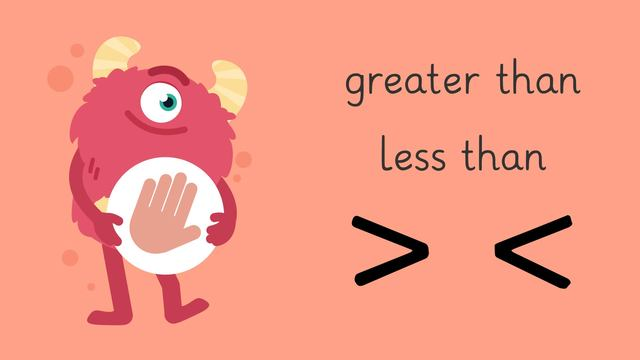# Greater Than/Less Than — Let's Practice!Rating

Ø 5.0 / 1 ratings
The authorsTeam Digital

### TranscriptGreater Than/Less Than — Let's Practice!

Razzi says get these items ready (...) Because today we're going to practice... Greater Than/Less Than It's time to begin! We compare numbers by talking about how big or small they are with the words like greater than, or less than. We always read number sentences from LEFT to RIGHT. Take a look at these numbers. Is twenty-five GREATER THAN or LESS THAN forty-five? Twenty-five is smaller than forty-five, so... twenty-five is LESS THAN forty-five. Let's tackle the next problem! Compare these numbers. Is thirty-six GREATER THAN or LESS THAN twenty-eight? Thirty-six is bigger than twenty-eight, so... thirty-six is GREATER THAN twenty-eight. Let's tackle the next problem! Take a look at these numbers. Is Fifty-seven GREATER THAN or LESS THAN sixteen? Fifty-seven is bigger than sixteen, so... Fifty-seven is GREATER THAN sixteen. Let's tackle the last problem! Is twenty-four GREATER THAN or LESS THAN sixty-eight? Twenty-four is smaller than sixty-eight, so... Twenty-four is LESS THAN sixty-eight. Razzi had so much fun practicing with you today! See you next time!

## Greater Than/Less Than — Let's Practice! exercise

Would you like to apply the knowledge you’ve learned? You can review and practice it with the tasks for the video Greater Than/Less Than — Let's Practice!.
• ### Is 33 greater than or less than 44?

Hints

We always read number sentences from left to right.

Is 33 smaller or bigger than 44?

Solution

33 < 44
33 is less than 44

• ### Is the number greater than or less than 57?

Hints

These are the signs used to show greater than and less than.

We always read number sentences from left to right.

23 < 57
This number sentence reads 23 is less than 57.

Solution

23 < 57
23 is less than 57

82 > 57
82 is greater than 57

17 < 57
17 is less than 57

73 > 57
73 is greater than 57

• ### Is the number greater than or less than 76?

Hints

We always read number sentences from left to right.

These are the signs used to show greater than and less than.

76 > 46
This number sentence reads 76 is greater than 46.

Solution

76 > 46
76 is greater than 46

76 < 89
76 is less than 89

76 < 94
76 is less than 94

76 > 32
76 is greater than 32

• ### Complete the word sentence below each number sentence.

Hints
• Read the number sentences from left to right.
• The bigger number is greater than the smaller number.
• The smaller number is less than the bigger number.
• In the first question, which number is bigger: 27 or 15?
• The bigger number is greater than the smaller number.
Solution

27 > 15
27 is greater than 15

19 < 91
19 is less than 91

51 < 72
51 is less than 72

46 < 64
46 is less than 64

75 > 38
75 is greater than 38

• ### Is 11 greater than or less than 7?

Hints

Is 11 bigger than or smaller than 7?

• Use your fingers to count.
• Do you say 7 or 11 first?
• Is 11 greater than or less than 7?
• Is 11 > 7 or is 11 < 7?
Solution

11 > 7
11 is greater than 7

• ### Complete the number sentence.

Hints

We always read number sentences from left to right.

• If a number is greater than another number, it is bigger than the other number.
• If a number is less than another number, it is smaller than the other number.
Solution

100 < 200
100 is less than 200

100 > 20
100 is greater than 20

25 < 50
25 is less than 50

50 < 100
50 is less than 100